4.4. The spatial distribution of X-ray emission

Early Uhuru observations indicated that the X-ray sources were centered on the cluster center, as determined from the galaxy distribution, or on an active galaxy in the cluster (Bahcall, 1977a). In many of the X-ray clusters, the cluster center also corresponds with the position of a cD or other central dominant galaxy.

The earliest X-ray observations showed that the X-ray sources in clusters were extended. With the nonimaging proportional counters used in these observations, the spatial resolution of the detectors was usually determined by mechanical collimators in front of the detectors and was therefore relatively crude (generally an angular resolution of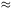1°). Since this is comparable to the sizes of the X-ray emission regions in the nearest clusters, the distribution of emission could not generally be observed in any detail. Only estimates of the size could be determined by convolving a model for the distribution of the emission with the resolution of the detector and comparing the result to the observations.

Lea et al. (1973), Kellogg and Murray (1974), and Abramopoulos and Ku (1983) derived sizes for cluster X-ray sources, assuming that the gas had the density distribution given by the King approximation to a self-gravitating isothermal sphere (equations 2.9, 2.13)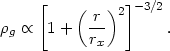(4.5)

Here,g is the gas density and rx is the X-ray core radius. While this model is not physically consistent (see Section 5.5.1), it does provide a convenient fitting form for comparison to the galaxy distribution, which is often fit by the same function. Values of rx were derived from the X-ray distribution in nine clusters by Lea et al. (1973) and Kellogg and Murray (1974). More recently, equation (4.5) has been fit to the distribution of X-ray emission from 53 clusters detected in an extensive survey of clusters with the Einstein X-ray observatory (Abramopoulos and Ku, 1983; Table 2). In general, these studies found that the X-ray core radii were significantly larger than the core radii of the galaxies (Section 2.7). These results were fairly uncertain because of the difficulties in determining either of these radii accurately.

More physically consistent hydrostatic models for the intracluster gas have also been used to fit the observed X-ray distributions (Lea, 1975; Gull and Northover, 1975; Cavaliere and Fusco-Femiano, 1976; Bahcall and Sarazin, 1977; Cavaliere, 1980). One model that has been used extensively is the hydrostatic isothermal model for the intracluster gas (Cavaliere and Fusco-Femiano, 1976, 1981; Bahcall and Sarazin 1977, 1978; Sarazin and Bahcall, 1977; Gorenstein et al., 1978; Jones and Forman, 1984; Section 5.5.1). In this model, both the galaxies and the intracluster gas are assumed to be isothermal, bound to the cluster, and in equilibrium. The galaxies are assumed to have an isotropic velocity dispersion. However, the gas and galaxies are not assumed to have the same velocity dispersion; the square of the ratio of the galaxy-to-gas velocity dispersions is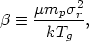(4.6)

where µ is the mean molecular weight in amu, mp is the mass of the proton,r is the one-dimensional velocity dispersion, and Tg is the gas temperature. Then, the gas and galaxy densities vary asggal. If the galaxy distribution is taken to be a King analytical form for the isothermal sphere (equation 2.13), then the X-ray surface brightness Ix(b) at a projected radius b varies as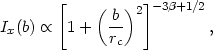(4.7)

where rc is the galaxy core radius (Section 2.7). The self-gravitating isothermal model (equation 4.5) has the same surface brightness distribution if one makes the replacements= 1 and rc = rx. These and other models for the distribution of the intracluster gas will be discussed in detail in Section 5.5.

The earlest determinations of the extent of the intracluster gas used low spatial resolution proportional counters. Observations with somewhat higher spatial resolution were made using detectors with modulation collimators (Schwarz et al., 1979) or one-dimensional imaging detectors (Gorenstein et al., 1973). Some clusters were found to contain compact X-ray sources associated with the central dominant galaxies in the cluster (Schnopper et al., 1977), such as NGC1275 in the Perseus cluster (Wolff et al., 1974, 1975, 1976; Cash et al., 1976; Malina et al., 1976; Helmken et al., 1978). In Perseus, this point source contributes20-25% of the X-ray luminosity at moderate photon energies (keV); at very high energies20 keV the point source is dominant (Primini et al., 1981; Rothschild et al., 1981). The X-ray surface brightness of the Perseus cluster was shown to be extended in the east-west direction (Wolff et al., 1974, 1975, 1976; Malina et al., 1976; Cash et al., 1976); this is the same direction as the line of bright galaxies seen optically in this L cluster. Later high resolution observations of the center of the cluster by Branduardi-Raymont et al. (1981) show a smaller elongation. A similar but smaller elongation was observed in the X-ray emission from the Coma cluster (Johnson et al., 1979; Gorenstein et al., 1979).

The Einstein X-ray telescope has allowed much more accurate determinations of the distribution of the X-ray emitting gas in clusters, using the moderate resolution (1 arc min) Imaging Proportional Counter (IPC) or the High Resolution Imager (HRI,8 arc sec). Moderate resolution Einstein IPC images of the X-ray emission in 46 clusters have been fit by equation (4.7) (Jones and Forman, 1984). Because galaxy core radii, velocity dispersions, and X-ray temperatures are poorly determined for most of these clusters, both the core radius andwere derived from the observed X-ray surface brightness. Table 2 gives the X-ray luminosities (Lx), X-ray core radii rx, and temperature parametersderived by Jones and Forman (the entries without an *). Note that the X-ray luminosity is only for gas within a projected radius of 0.5 Mpc of the cluster center. Abramopoulos and Ku (1983) fit IPC observations of 53 clusters to equation (4.5); that is, they assumed= 1. Table 2 also contains the results of their fits (the entries marked with an *) for the clusters with X-ray detections which were not studied by Jones and Forman. The values of the total X-ray luminosities of Abramopoulos and Ku were converted to luminosities within 0.5 Mpc using their best fit X-ray distribution. Of course, the value offor all the Abramopoulos and Ku fits is listed as one. It is worth noting that in nearly every case where both Abramopoulos and Ku, and Jones and Forman studied the same cluster, Jones and Forman found best fit values ofwhich were much smaller than the= 1 assumed by Abramopoulos and Ku, and Jones and Forman could rule out the value= 1 with high statistical confidence. Thus the validity of the results of Abramopoulos and Ku is questionable because of the value ofassumed.

Figure 16 shows the X-ray surface brightness as a function of radius from the center of the X-ray emission (in minutes of arc) for A400, A2063, and A1795 from Jones and Forman (1984). The solid histogram is the data; the dots are the best fit using equation (4.6). About two-thirds of the clusters were fit quite well with this formula; however, one-third appeared to have an excess of emission near the cluster center. For those clusters with a central excess, the central surface brightness points were excluded for the fits given in Table 2. In Figure 16, A400 and A2063 were reasonably fit by equation (4.6), while A1795 was not. The second panel on A1795 shows the fit to equation (4.6) if the central several minutes of arc are ignored. Jones and Forman suggest that this excess central X-ray emission correlates with the radio luminosity of the cluster, and that the excess emission may be due to a cooling flow in the cluster core (Section 5.7). A1795 does in fact have a very large cooling flow (Table 4 below).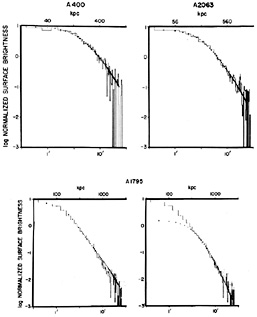Figure 16. The X-ray surface brightness of several clusters, as determined by the IPC on the Einstein satellite by Jones and Forman (1984). The surface brightness is normalized to its central value and is given as a function of the angular distance from the cluster center. The solid curves give the observed surface brightness, and the dots are the best fit using equation (4.7). The bottom two panels show the A1795 cluster with the inner eight data points either included in the fit (left) or removed (right). The improvement in the fit in the outer points when the inner regions are removed suggests excess emission in the center, due to a cooling flow.

A wide range of core radii (0.07 - 0.9h50-1 Mpc) were derived by Jones and Forman. They found a strong anticorrelation between the presence of a dominant cluster galaxy in the core and the size of the X-ray core radius. (Unfortunately, Abramopoulos and Ku (1983) found the opposite effect with their sample of clusters observed with Einstein.) The average value offound by Jones and Forman from the X-ray distributions is= 0.65, which implies that the gas is considerably more extensively distributed than the mass in the cluster. Unfortunately, the average value ofdetermined by applying equation (4.6) to those clusters with measured velocity dispersions and X-ray temperatures is= 1.1. It is not clear whether this discrepancy results from errors in the measured cluster properties, velocity anisotropies, or a failure of the isothermal model (see Section 5.5.1).

 References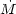Cluster (M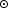/ yr) X-ray Optical A85 120 25 13,4 A262 28 25 14 A400 2 25 A426 Perseus 300 15,4 A496 200 22,20 13,7,4 A576 40 26,23 A978 500 13 A1060 6 25 A1126 500 13,14 A1795 400 25,20 13,4 A1983 7 25 A1991 115 25 A2029 250 25,20 4 A2052 120 13,14,5 A2063 26 25 A2107 18 25 A2142 28 16 4 A2199 110 25,20 4 A2319 75 25,26 4 A2415 15 25 A2626 10 25 A2657 36 25 A2670 78 25 SC0107-46 4 25 AWM7 40 3 3A0335+096 280 24,20 MKW4 7 18,3 SC0745-191 1000 9 9 M87/Virgo 3-20 10,11,2,17,20 12 Centaurus 22 19 8 MKW3s 100 3 AWM4 25 3 SC1842-63 3 25 SC2059-247 500 27 References 1 Canizares (1981) 2 Canizares et al. (1979,1982) 3 Canizares et al. (1983) 4 Cowie et al. (1983) 5 Demoulin-Ulrich et al. (1984) 6 Fabian et al. (1981a) 7 Fabian et al. (1981b) 8 Fabian et al. (1982a) 9 Fabian et al. (1985) 10 Fabricant et al. (1980) 11 Fabricant and Gorenstein (1983) 12 Ford and Butcher (1979) 13 Heckman (1981) 14 Hu et al. (1985) 15 Kent and Sargent (1979) 16 Lea et al. (1981) 17 Lea et al. (1982) 18 Malumuth and Kriss (1986) 19 Matilsky et al. (1985) 20 Mushotzky (1984) 21 Mushotzky et al. (1981) 22 Nulsen et al. (1982) 23 Rothenflug et al. (1984) 24 Schwartz et al. (1980b) 25 Stewart et al. (1984b) 26 White and Silk (1980) 27 White et al. (1981a)

While in most clusters the X-ray emission was found to be as broadly distributed as the galaxy distribution, an exception was Virgo/M87. Here the soft X-ray emission comes from a small region around M87, while the galaxy core radius of this irregular cluster, although hard to define, is certainly much larger. While early observations suggested the existence of weaker hard X-ray emission originating from a larger region of the cluster (Davison, 1978; Lawrence, 1978), recent observations indicate that this emission actually is due to the nucleus of M87 (Lea et al., 1981, 1982).

The thermal X-ray emission from intracluster gas is proportional to the emission integral EI (equation 4.3), while the mass of intracluster gas is proportional tonpdV. All other things being equal, the mass of the intracluster gas is then Mg(Lx rx3)1/2. Thus, if the size and distribution of the X-ray emitting gas can be determined from the X-ray surface brightness, the mass in intracluster gas can be estimated. Unfortunately, the estimate is very uncertain because the X-ray emission falls off rapidly as the density decreases in the outer parts of the cluster, where a large fraction of the mass of the intracluster gas may be located. Early estimates of the mass of the intracluster gas based on the self-gravitating isothermal model (equation 4.7) were given in Lea et al. (1973) and Kellogg and Murray (1974). They found that the total mass of intracluster gas determined from the X-ray observations is similar to or somewhat larger than the total mass of the galaxies in the cluster estimated from their total luminosity and a typical galaxy mass-to-light ratio. However, based on these mass estimates, the intracluster gas is still only 5-15% of the total virial mass of the cluster, and thus the discovery of the intracluster gas has not resolved the missing mass problem in clusters (Section 2.8). Table 2 gives the intracluster gas masses (here within 3.0 Mpc radius of the cluster center) from the Einstein observations of Abramopoulos and Ku (1983), and Jones and Forman (1984). Recall that Abramopoulos and Ku assumed that= 1, which is generally larger than the best fit values from Jones and Forman. Reducing the values ofgreatly increases the gas mass for a fixed X-ray luminosity, and the gas mass actually diverges at large radii for1. Thus the gas masses found by Jones and Forman are generally considerably larger than those found by Abramopoulos and Ku or the earlier studies. As similar result, using the HEAO-1 A-2 X-ray spectra as well as the Einstein X-ray images, was found by Henriksen and Mushotzky (1985). Because of uncertainities in the gas and virial mass densities at large radii, it is difficult to give an accurate determination of the mass fraction of the intracluster gas. It does appear likely that, on average, at least 10% of the virial mass must be in the form of intracluster gas. The fraction of the total mass in intracluster gas could in some cases be as high as 30-60%, if the intracluster gas is as extended as the results of Henriksen and Mushotzky suggest.